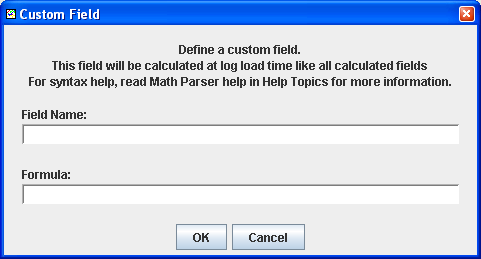Performance, Drivability & Efficiency. Simplified! MegaLogViewer HDHeavy Duty - Premium Edition of MegaLogViewer
 Try MegaLogViewer HD Free Now! Register MegaLogViewer HD  Now! MegaLogViewer MS User? Upgrade
<< Home

# MegaLogViewer Formulas

MegaLogViewer uses a custom built math parser that gives the end user a great deal of power to add their own custom fields or filters. The Math Parser executes logical evaluation and equation execution. You can reference fields to be used as input to your custom calculations. Here are some samples and explanations:
Custom Fields
Simple Calculations
Logical Conditions & Expressions
In-Line Evaluation

Custom Fields:
MegaLogViewer supports custom fields, that is fields calculated using a formula defined by the user. The formula can be as complex as you wish, following the guidelines on this page.
It is recommended that this is used for adding your own fields as opposed to editing the properties files. I discourage editing of the properties files as your changes will be over written on the next update. If you come up with formulas you feel many would beefit from or find a need to edit the properties file(s), please contact EFI Analytics. We can incorporate it in the next release for all.

To create a custom field:
From the Menu bar select:
"Calculated Fields" -> "Custom Fields" -> "Add Custom Field"• Enter a Name for your custom field. The name must be unique and is case sensitive. Example: deltaRPM
• Enter the formula as outlined below. Example: [RPM]-[RPM-1]

Simple calculations:
deltaRPM=[RPM]-[RPM-1]
interpreted as:
deltaRPM = (Current RPM)-(RPM 1 record back)

You can reference previous records by incuding the -x inside the []. As seen above [RPM] references the current RPM, where [RPM-1] now references the RPM 1 record previous. You can reference back further, i.e [RPM-2] will reference 2 records back, [RPM-3] is 3 back, and so on. All references are relative.
Operators:

• +
• -
• /
• *
• ^
• ~
• &
• |
• %
• >>
• >>>
• <<
• !
Math is executed from left to right, operation hierarchy is not recognized. If you need to
insure execution order parenthesis are honored.
calcValu = [RPM]/([RPM]*1.2)
the [RPM]*1.2 will be executed first.

Supported Advanced Math functions are:

 Function Definition Usage Smoothing Smooths a field by averaging over the smoothingFactor number of records. smoothBasic( field, smoothingFactor) sine Standard Sine of a value. sin(val) cosine Standard Cosine of a value. cos(val) arcsine Standard arcsine of a value. asin(val) arccosine Standard arccosine of a value. acos(val) tangent Standard Tangent of a value. tan(val) arc tangent Standard Arc Tangent of a value. atan(val) square root Standard Square Root, same as pow(val, 0.5) of a value. sqrt(val) absolute Changes any negative values to the same magnitude in the positive direction. abs(val) log Natural log of a value. log(val) log base 10 Base 10 log of a value of a value. log10(val) recipricol The recipricol of a value, or 1/val recip(val) exponent Exponent of a value. pow(val, exponent) round Rounds the value of a value to the nearest integer value. round(val) floor Returns the largest (closest to positive infinity) integer value that is greater than or equal to the argument. floor(val) ceiling Returns the smallest (closest to negative infinity) integer value that is greater than or equal to the argument. ceil(val) exponent Returns Euler's number e raised to the power of a double value. exp(val) isNaN Checks the output of an expression to result in a invalid number isNaN(val) smoothFiltered Smooth a field using a matrix filter for less lag smoothFiltered(val) accelHp Calculates HP based on Acceleration rate. accelHp(     velocity (MPH),     deltaVelocity(MPH),     deltaTime(s),     weight(lb) ) Aero Drag Calculates the Aerodynamic Drag aerodynamicDragHp(     velocity (m/s),     airDensity (kg/m^-3),     dragCoefficent,     frontalArea (m^2) ) Rolling Drag Calculates estimated rolling resistence rollingDragHp(     speed (MPH),     tirePressure (psi),     weight (lbs) )

To use these Math Functions:
result = func(expression)
The input Expression can be as complex or simple as needed.

Example:
calcVal = abs([RPM]-[RPM-5])
the calcVal will alway represent the absolute value difference between the current RPM and RPM 5 records earlier.
or
calcVal = smoothBasic([AFR], 5)

Logical Conditions
Conditions will result in a true or false state. These can be used in several places including Custom Filters in VE Analyze.
[RPM]>2000
interpreted as:
true when RPM is greater than 2000, false if 2000 RPM or below

Any form of complex math expresion can be used on each side of the operator.
Supported comparators:

• ==
• !=
• >=
• <=
• >
• <
You can also use compound conditions separated by:
• && or AND
• || or OR
Example:
[RPM] > 2000 || [RPM] < 1000
This would return false for any RPM value from 1000 to 2000, otherwise it will return true.

In-line evaluation
In some instances you may want different calculations performed based on a condition. this can be performed with an in-line evaluation
output = {condition} ? {true expression} : {false expression}

Example:
calcVal = [RPM] > 1000 ? [RPM] : 0
this will provide RPM for anything over 1000, anything under will be 0
Naturally You can get as fancy as needed in the condition, true expression and false expression. some good examples of rather complex calculations can be found in the MSGraph.properties file for MegaLogViewer. Checkout the calculations for "Instant MPG" or HP